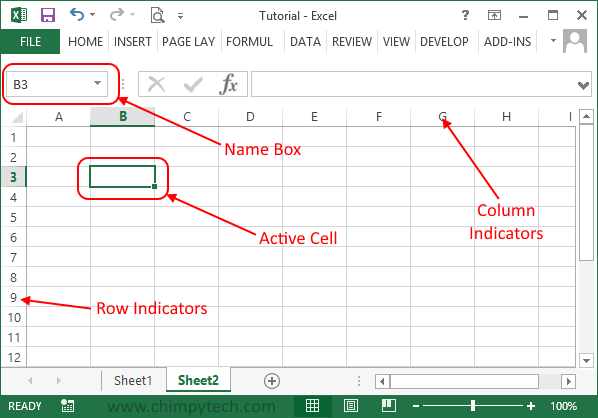# Excel Rows and Columns Explained

Now that you are able to Open, Close, and manipulate your Excel workbooks and Worksheets, it’s time to have a look at how a worksheet is constructed.

Each Excel Worksheet is divided into a number of rows and columns, and the intersection of each row and column is known as a cell.In Excel 2013 each sheet can have 16384 columns (labelled from A to XFD) and 1048576 rows (numbered from 1 to 1048576).

Where a row and column meet, each box is known as a cell, in the picture above cell B3 has been selected to make it the active cell, which is indicated by the green border. There is also a section in the menu area known as the Name Box, this also shows which cell is active, this can be useful if the active cell is currently off screen.

Each cell can contain either:

• A number, which may be used in the formulas contained in another cell.
• Some text which can be used to describe the contents of other cells.
• A formula which can be used to manipulate data or other cells within the Worksheet